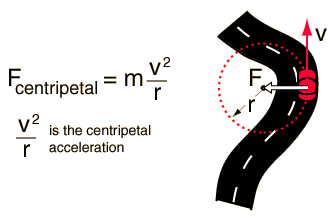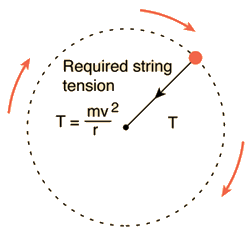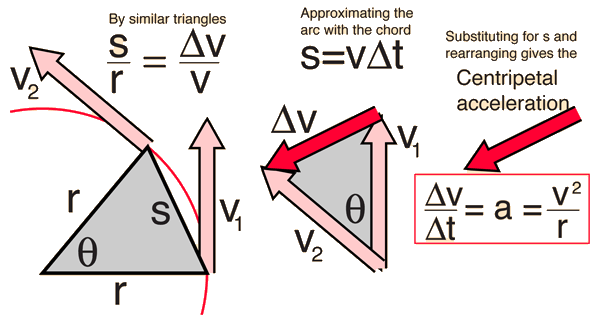# Centripetal Force

Any motion in a curved path represents accelerated motion, and requires a force directed toward the center of curvature of the path. This force is called the centripetal force which means "center seeking" force. The force has the magnitudeSwinging a mass on a string requires string tension, and the mass will travel off in a tangential straight line if the string breaks.

The centripetal acceleration can be derived for the case of circular motion since the curved path at any point can be extended to a circle.Note that the centripetal force is proportional to the square of the velocity, implying that a doubling of speed will require four times the centripetal force to keep the motion in a circle. If the centripetal force must be provided by friction alone on a curve, an increase in speed could lead to an unexpected skid if friction is insufficient.

### Calculation

 Centripetal force on banked highway curve
Index

Example with mass on string

 HyperPhysics***** Mechanics ***** Rotation R Nave
Go Back

# Centripetal Force Calculation

## Centripetal force = mass x velocity2 / radiusNote that the conditions here assume no additional forces, like a horizontal circle on a frictionless surface. For a vertical circle, the speed and tension must vary.

Any of the data values may be changed. When finished with data entry, click on the quantity you wish to calculate in the formula above. Unit conversions will be carried out as you enter data, but values will not be forced to be consistent until you click on the desired quantity.

Calculation for:
Radius r = m = ft
Mass = m=kg = slugs
Weight = W=N = lbs
Velocity = v=m/s = ft/s
or in common highway speed units,
velocity = km/h = mi/h

Centripetal force= F=N = lbs

### Discussion of concept

Index

 HyperPhysics***** Mechanics ***** Rotation R Nave
Go Back

# Centripetal Acceleration

The centripetal acceleration expression is obtained from analysis of constant speed circular motion by the use of similar triangles. From the ratio of the sides of the triangles:For a velocity of m/s and radius m, the centripetal acceleration is m/s².
Note that if the velocity is doubled to m/s at the same radius, the acceleration is quadrupled to m/s2.
Index

 HyperPhysics***** Mechanics ***** Rotation R Nave
Go Back